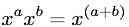Equations > Algebra > Exponents/Powers > Addition of Exponents Rule

### Addition of Exponents RuleLatex Code:

MathML Code:

 ${x}^{a}{x}^{b}={x}^{\left(a+b\right)}$

MathType 5.0: